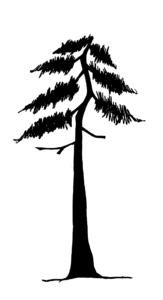# 2.8: Summary Questions

1. Determine the total heights of the trees illustrated below. Pay attention to the horizontal distances and slope scale used for each.2. How should the total height of the flat-topped tree below be determined?3. Calculate the height of the leaner tree below.1. A.(%slope)(137+11)

rise (height)= 74 feet

I.B.(97+16)

= 113 feet

I.C.(107+12)

= 143 feet

I.D.(Tslope)(101+8)

rise (height) = 109 feet

I.E.(90+10)

= 50 feet

2. The top of the tree will have to be reconstructed because of the broken top. This can be done by using a neighboring tree that is similar in size and taper as a reference.

3. a. The rise of the fall line from the top of the tree to the ground =(Tslope)(48+8)

= 56 feet

b. The horizontal distance from the base of the tree to the fall line is 54 feet. Thus we have two sides of the right triangle.

Using the Pythagorean Theorem to determine the tree length (hypotenuse):

a2 + b2 = c2 where:

562 + 542 = c2

6052 = c278 feet